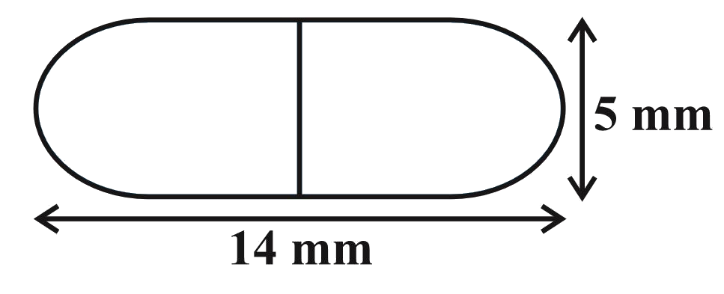# Ex.13.1 Q6 Surface Areas and Volumes Solution - NCERT Maths Class 10

Go back to  'Ex.13.1'

## Question

A medicine capsule is in the shape of cylinder with two hemispheres stuck to each of its ends (see Fig. 13.10). The length of the entire capsule is $$14 \,\rm{mm}$$ and the diameter of the capsule is $$5 \,\rm{mm}$$. Find its surface area.Video Solution
Surface Areas And Volumes
Ex 13.1 | Question 6

## Text Solution

What is known?

A medicine capsule is in the shape of a cylinder with two hemispheres stuck to its ends. The length of the entire capsule $$=14 \,\rm{mm}$$, diameter of the capsule $$=5 \,\rm{mm}$$.

What is the unknown?

The surface area of the capsule.

Reasoning:

Since the capsule is in shape of a cylinder with $$2$$ hemispheres stuck to its ends,

Diameter of the capsule $$=$$ diameter of its cylindrical part $$=$$ diameter of its hemispherical part.

From the figure, it’s clear that the capsule has the curved surface of two hemispheres and the curved surface of a cylinder.

Surface area of the capsule $$= 2 \times$$ CSA of hemispherical part $$+$$ CSA of cylindrical part

We will find the surface area of the capsule by using formulae;
CSA of the hemisphere  $$= 2\pi {r^2}$$
where $$r$$ is the radius of the hemisphere
CSA of the cylinder $$= 2\pi rh$$
where $$r$$ and $$h$$ are radius and height of the cylinder respectively.

Length of the cylindrical part $$=$$ Length of the capsule $$- 2 \times$$ radius of the hemispherical part

Steps:

Diameter of the capsule,  $$d = 5 \rm mm$$
Radius of the hemisphere, \begin{align}r = \frac{d}{2} = \frac{5}{2} \rm mm\end{align}
Radius of the cylinder,  \begin{align}r = \frac{5}{2} \rm mm\end{align}
Length of the cylinder = Length of the capsule$$- 2 \times$$ radius of the hemisphere

$h = 14 \rm mm - 2 \times \frac{5}{2}mm = 9mm$

Surface area of the capsule$$- 2 \times$$ CSA of hemispherical part $$+$$ CSA of cylindrical part

\begin{align}& = 2 \times 2\pi {r^2} + 2\pi rh\\ &= 2\pi r\left( {2r + h} \right)\\ &= \begin{bmatrix} 2 \times \frac{{22}}{7} \times \frac{5}{2} \rm {mm} \times \\ \left( {2 \times \frac{5}{2}\rm {mm} + 9\rm {mm}} \right) \end{bmatrix} \\&= \frac{{110}}{7} \rm{mm} \times 14{mm}\\&= 220 \rm {m{m^2}}\end{align}

Learn from the best math teachers and top your exams

• Live one on one classroom and doubt clearing
• Practice worksheets in and after class for conceptual clarity
• Personalized curriculum to keep up with school